# Artificial Neural Networks n Artificial Neural Networks are

• Slides: 20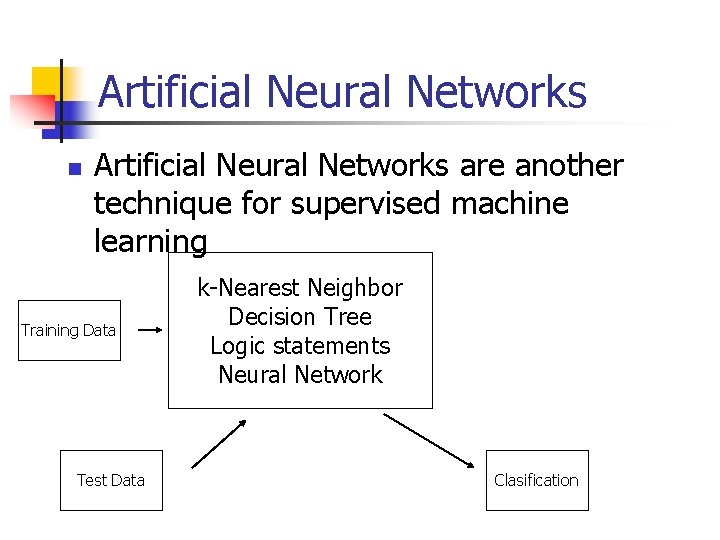Artificial Neural Networks n Artificial Neural Networks are another technique for supervised machine learning Training Data Test Data k-Nearest Neighbor Decision Tree Logic statements Neural Network Clasification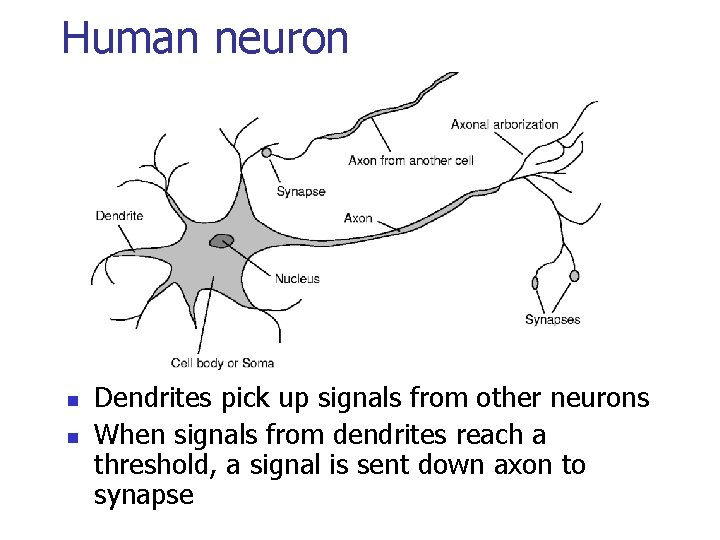Human neuron n n Dendrites pick up signals from other neurons When signals from dendrites reach a threshold, a signal is sent down axon to synapseConnection with AI n Most modern AI: n n Implementing neurons in a computer n n “Systems that act rationally” “Systems that think like humans” Why artificial neural networks then? n n “Universal” function fitter Potential for massive parallelism Some amount of fault-tolerance Trainable by inductive learning, like other supervised learning teachniques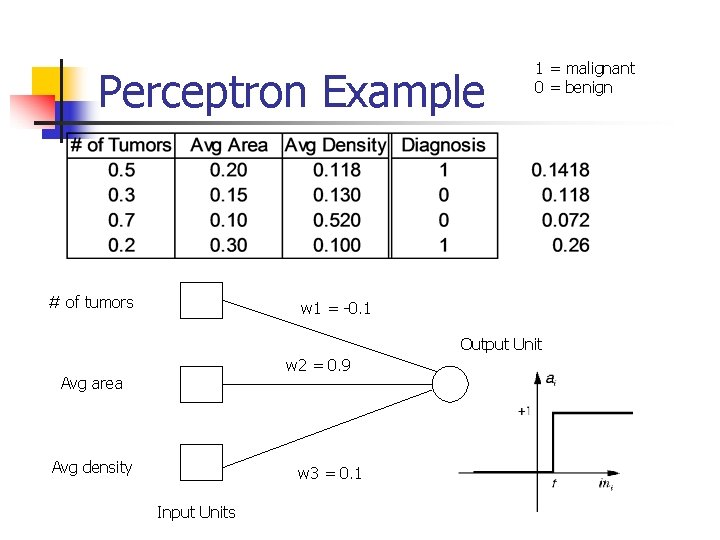Perceptron Example # of tumors 1 = malignant 0 = benign w 1 = -0. 1 Output Unit w 2 = 0. 9 Avg area Avg density w 3 = 0. 1 Input Units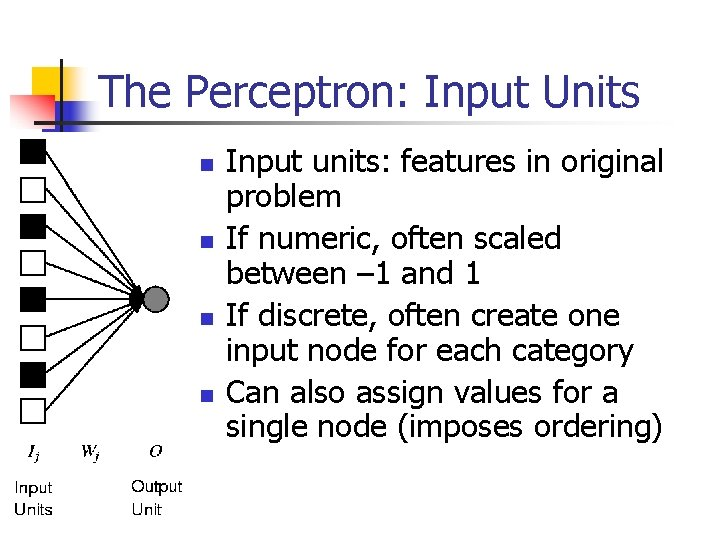The Perceptron: Input Units n n Input units: features in original problem If numeric, often scaled between – 1 and 1 If discrete, often create one input node for each category Can also assign values for a single node (imposes ordering)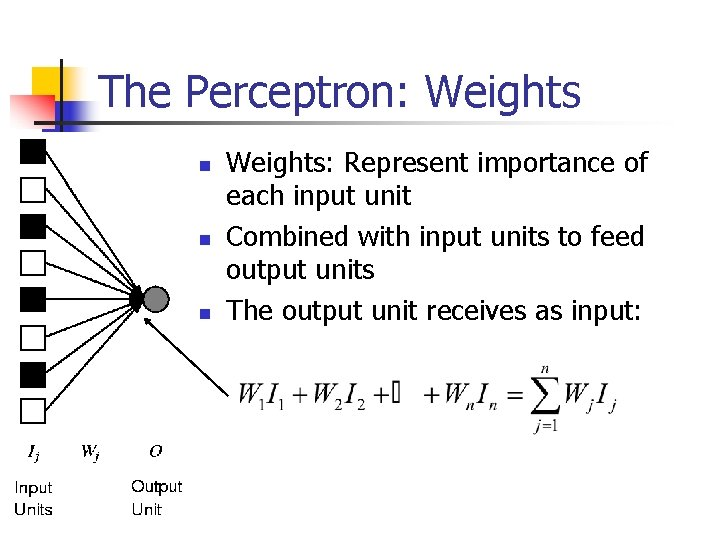The Perceptron: Weights n n n Weights: Represent importance of each input unit Combined with input units to feed output units The output unit receives as input:The Perceptron: Output Unit n n The output unit uses an activation function to decide what the correct output is Sample activation function: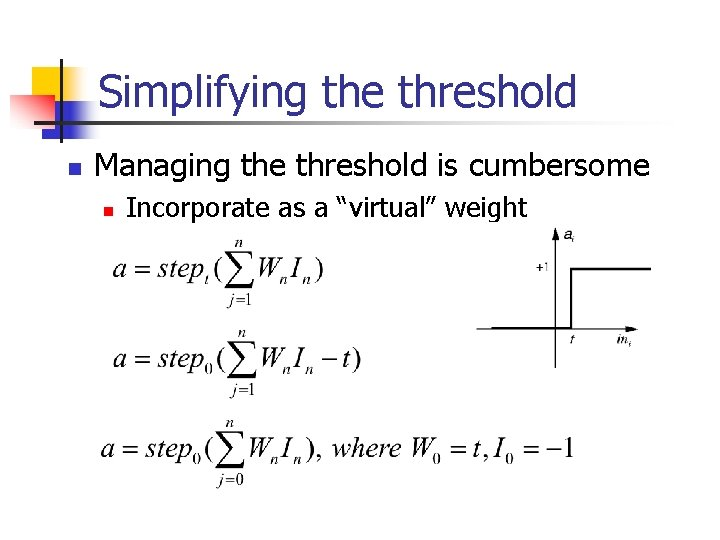Simplifying the threshold n Managing the threshold is cumbersome n Incorporate as a “virtual” weight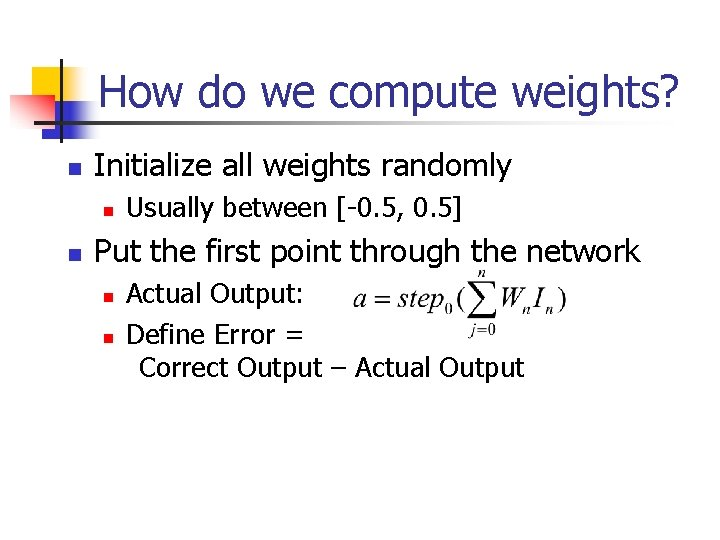How do we compute weights? n Initialize all weights randomly n n Usually between [-0. 5, 0. 5] Put the first point through the network n n Actual Output: Define Error = Correct Output – Actual Output1 = malignant 0 = benign Perceptron Example # of tumors w 1 = -0. 3 w 0 = 0 Output Unit w 2 = -0. 2 Avg area Avg density w 3 = 0. 4 Input Units Actual Output = 0 Correct Output = 1 Error = 1 – 0 = 1 If input is positive, want weight to be more positive If input is negative, want weight to be more negativeThe Perceptron Learning Rule: How do we compute weights? n Put the first point through the network n n n Actual Output: Define Error = Correct Output – Actual Output Update all weights: a = learning rate Repeat with all points, then all points again, and again, until all correct or stopping criterion reachedCan appropriate weights always be found? n ONLY IF data is linearly separableWhat if data is not linearly separable? Neural Network. O Vj n n Each hidden unit is a perceptron The output unit is another perceptron with hidden units as input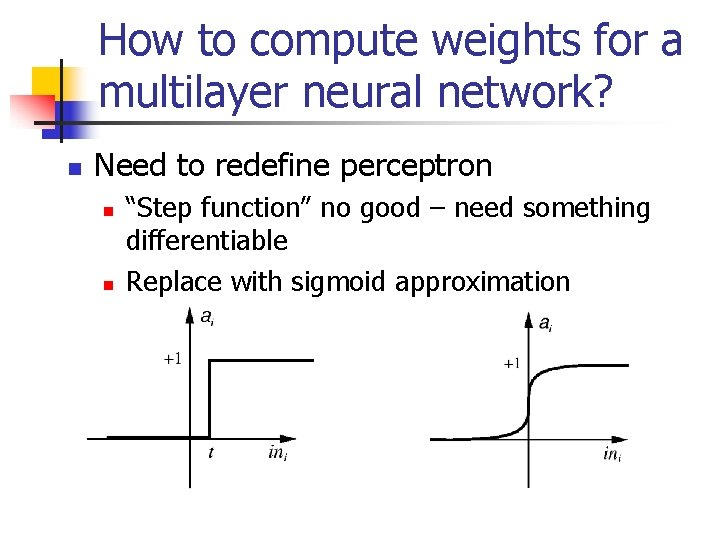How to compute weights for a multilayer neural network? n Need to redefine perceptron n n “Step function” no good – need something differentiable Replace with sigmoid approximationSigmoid function n Good approximation to step function As b infinity, sigmoid step We’ll just take b = 1 for simplicity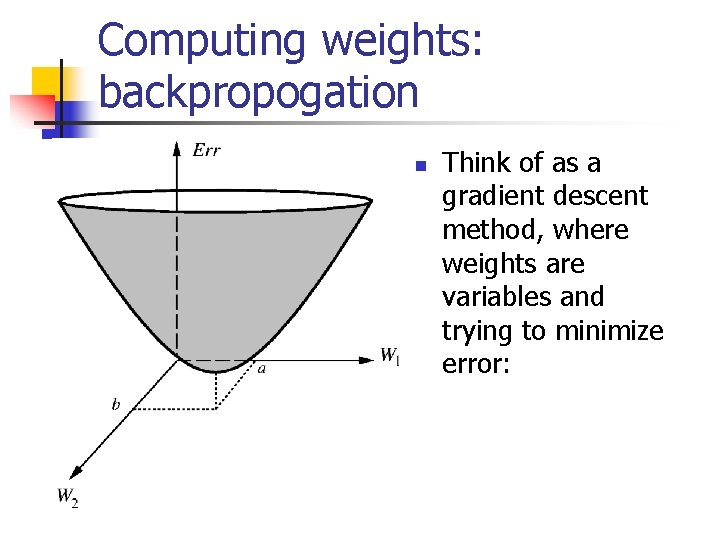Computing weights: backpropogation n Think of as a gradient descent method, where weights are variables and trying to minimize error:Minimize squared errors n For all training points, let n n Tp = correct output Op = actual output Want to minimize error Work with one point at a time, and move weights in direction to reduce error the mostExpand (drop the p for simplicity) n Direction of most rapid positive rate of change (gradient) is given by partial derivative Update rule for hidden layer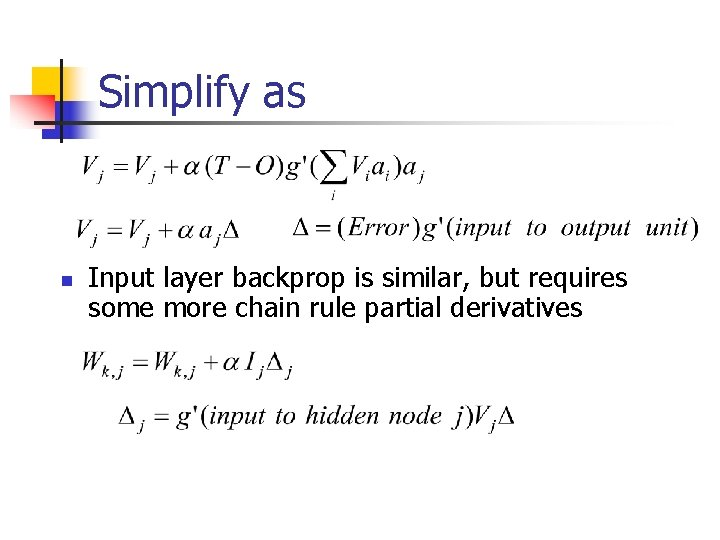Simplify as n Input layer backprop is similar, but requires some more chain rule partial derivatives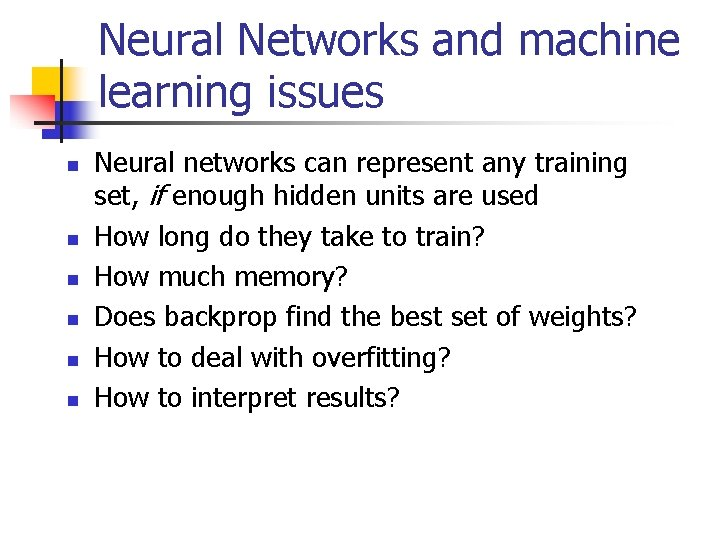Neural Networks and machine learning issues n n n Neural networks can represent any training set, if enough hidden units are used How long do they take to train? How much memory? Does backprop find the best set of weights? How to deal with overfitting? How to interpret results?